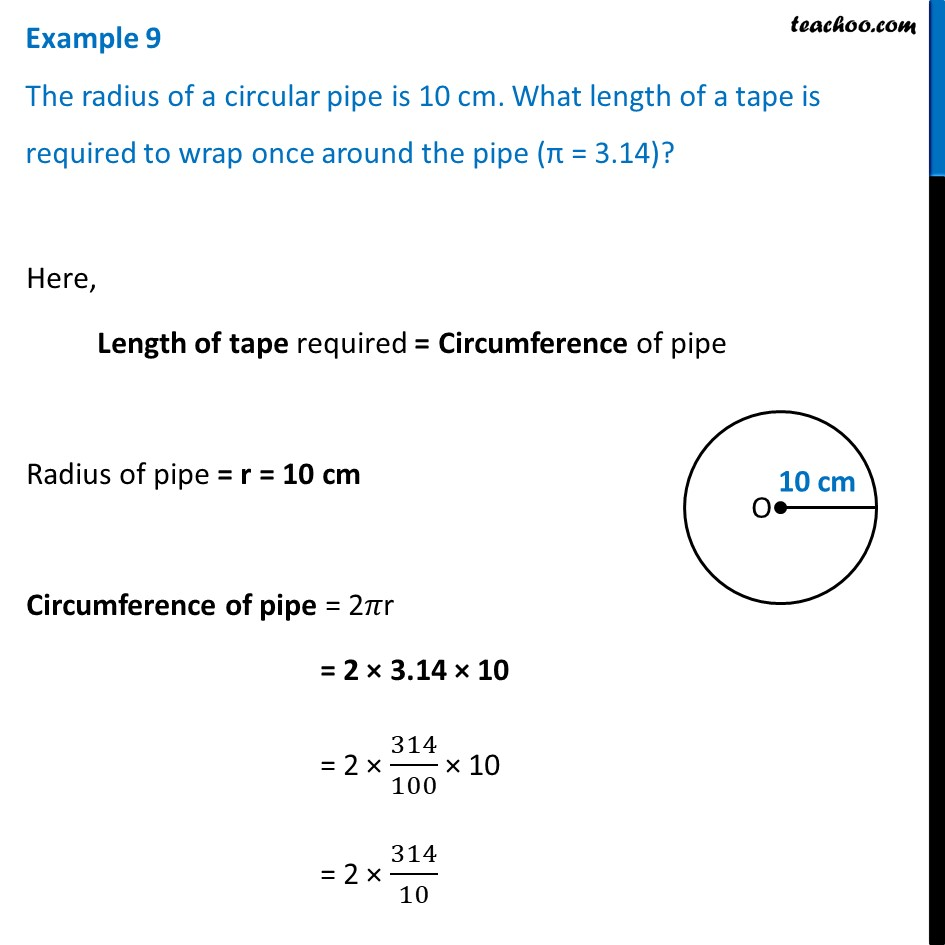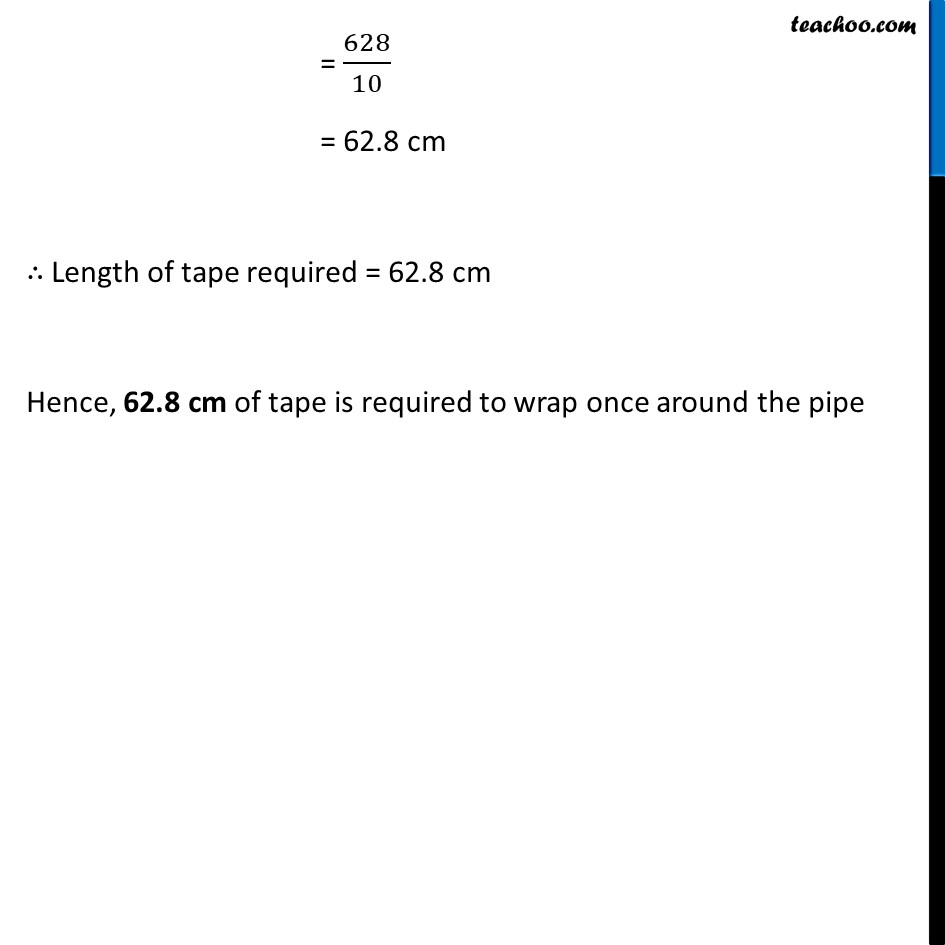Examples

Chapter 9 Class 7 Perimeter and Area
Serial order wiseLearn in your speed, with individual attention - Teachoo Maths 1-on-1 Class

### Transcript

Example 9 The radius of a circular pipe is 10 cm. What length of a tape is required to wrap once around the pipe (π = 3.14)?Here, Length of tape required = Circumference of pipe Radius of pipe = r = 10 cm Circumference of pipe = 2𝜋r = 2 × 3.14 × 10 = 2 × 314/100 × 10 = 2 × 314/10 = 628/10 = 62.8 cm ∴ Length of tape required = 62.8 cm Hence, 62.8 cm of tape is required to wrap once around the pipe